Next: Stability and Mesh Size Up: Review of the Cohesive/Volumetric Previous: Formulation   Contents

## Finite Element Implementation

The implementation of the CVFE scheme relies on the following form of the principle of virtual work: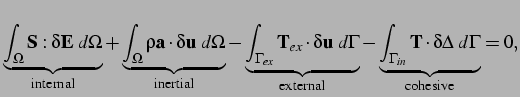(2.6)

where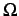is the undeformed domain,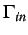denotes the interior cohesive'' boundary along which the cohesive tractions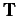act, and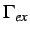corresponds to the part of the exterior boundary along which the external tractions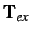are applied.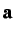and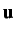denote the acceleration and displacement fields, respectively.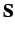is the second Piola-Kirchoff stress tensor and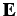, the Lagrangian strain tensor, which is related to the displacement field through(2.7)

Nonlinear kinematics is used in this study to account for the possible large rotations present in the structure due to the fracture process. The expression (2.6) of the principle of virtual work is fairly conventional, except for the presence of the fourth term, which corresponds to the virtual work done by cohesive tractionfor a virtual separation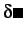.

The resulting semi-discrete finite element formulation can be expressed in the following matrix form: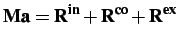(2.8)

where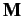is the lumped mass matrix,is the vector containing the nodal accelerations, and,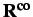and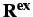respectively denote the internal, cohesive and external force vectors.

The time stepping scheme is based on the classical explicit second-order central difference scheme (Belytschko et al., 1976):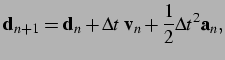(2.9)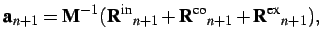(2.10)(2.11)

where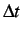is the time step and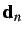denotes the nodal displacement vector at time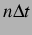.

The expression of the internal, cohesive and external force vectors can be found in Baylor (1997).While a variety of constitutive models can be used to characterize the response of the volumetric elements, we use, in this study, a simple linear isotropic relation between the second Piola-Kirchoff stressesand the Lagrangian strains: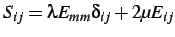(2.12)

where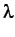and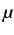are the Lame's constants.Next: Stability and Mesh Size Up: Review of the Cohesive/Volumetric Previous: Formulation   Contents
Mariusz Zaczek 2002-10-13